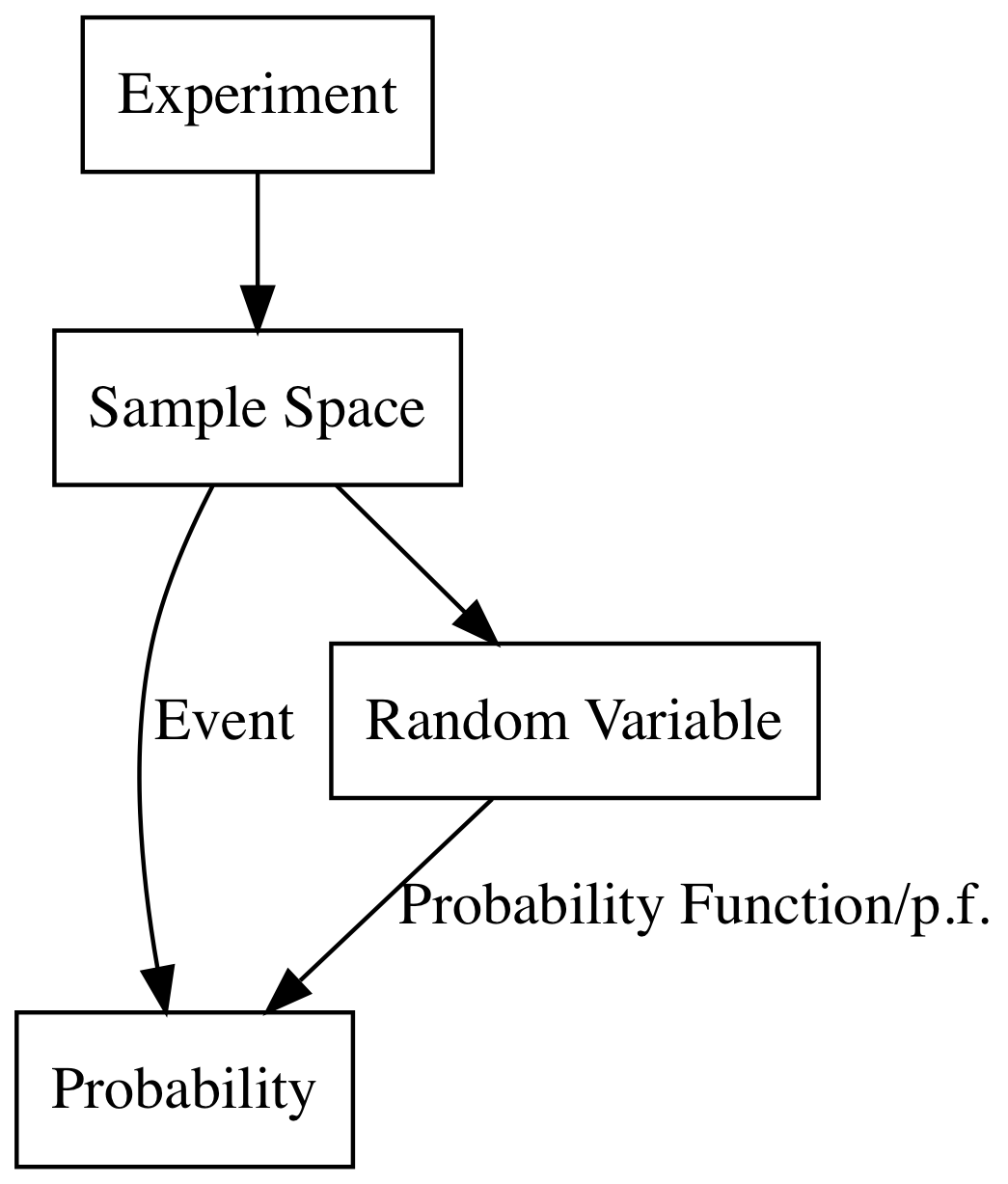## 随机变量 Random Variables

### 随机变量的定义 Definition of a Random Variable

Definition Random Variables:Let S be the sample space for an experiment.A real-valued fuction that is defined on S is call a random variable

“这个定义是个概率论非常关键的转折，从此我们从赌博走向了数学的康庄大道”

## 随机变量的分布 The Distribution of a Random Variable

$$Pr(X\in C)=Pr({s:X(s)\in C})$$

Definition Ditribution :Let X be a random variable.The distribution of X is the collection of all probabilities of the form $Pr(X\in C)$ for all sets C of real numbers such that ${X\in C}$ is an event

$$Pr(X=x)=\sum_{s\in{X(s)=x}}Pr(s)=\begin{pmatrix}10\\x\end{pmatrix}\frac{1}{2^{10}} \quad for\quad x=1,2\dots, 10$$

## 离散分布 Discrete Distribution

Definition Discrete Distribution/Random Variable:We say that a random variable $X$ has a discrete distribution or that $X$ is a discrete random variable if $X$ can take only a finite number k of different values $x_1,\dots , x_k$ or at most an infinite sequence of different values $x_1,x_2\dots$

Definition Probability Function/p.f./Support:If a random variable $X$ has a discrete distribution the probability function (abbreviated p.f.)of $X$ is defined as the function $f$ such that for every real number $x$ ,
$$f(x)=Pr(X=x)$$
the closure of the set ${x:f(x)>0}$ is called the support of (the distribution of)$X$

$$X(s)=\begin{cases} 1& \text{s为正面}\\ 0& \text{s为反面} \end{cases}$$

$$Pr(X=1)=Pr(s为正面)=0.6\\ Pr(X=0)=Pr(s为反面)=0.4$$

$$f(x)=\begin{cases} 0.6& \text{x=1}\\ 0.4& \text{x=0} \end{cases}$$

Let $X$ be a discrete random variable with p.f. $f$ ,If $x$ is not one of the possible values of $X$ ,then $f(x)=0$ also if the sequence $x_1,x_2,\dots$ include all the possible values of X then $\sum^{\infty}_{i=1}f(x_i)=1$

Theorem If $X$ has a discrete distribution,the probability of each subset $C$ of the real line can be determined from the relation:
$$Pr(X\in C)=\sum_{x_i\in C}f(x_i)$$## 均匀分布 Uniform Distributions(Discrete)

Definition Uniform Distribution on Integers: Let $a\leq b$ be integers.Suppose that the value of a random variable $X$ is equally likely to be each of the integers $a,\dots ,b$ .Then we say that $X$ has the uniform distribution on the integers $a,\dots,b$
Theorem If X has the uniform distribution on the integers $a,\dots ,b$ ,the p.f. of X is :
$$f(x)=\begin{cases} \frac{1}{b-a+1} &\quad \text{for x=a,\dots,b}\\ 0& \text{otherwise} \end{cases}$$

## 二项分布 Binormial Distributions

$$Pr(s_1)=p^{m}(1-p)^{N-m}$$

$$\begin{pmatrix}N\\m\end{pmatrix}$$

$$Pr(X=m)=\begin{pmatrix}N\\m\end{pmatrix}p^{m}(1-p)^{N-m}$$

$$Pr(X=x)=\begin{pmatrix}N\\x\end{pmatrix}p^{x}(1-p)^{N-x}$$

X的p.f. 是：

$$f(x)=\begin{cases} \begin{pmatrix}N\\x\end{pmatrix}p^{x}(1-p)^{N-x} &\quad \text{for x=0,1,\dots,N}\\ 0& \text{otherwise} \end{cases}$$

Definition Binomial Distribution/Random Variable:The distribution represeanted by the p.f.

$$f(x)=\begin{cases} \begin{pmatrix}N\\x\end{pmatrix}p^{x}(1-p)^{N-x} &\quad \text{for x=0,1,\dots,N}\\ 0& \text{otherwise} \end{cases}$$

is called the binomial distribution with parameters $n$ and $p$ .A random variable with this distribution is said to be a binomial random variable with parameters $n$ and $p$

## 总结

### 1 说点什么1 Followers

Most reacted commentRecent comment authorswpDiscuz